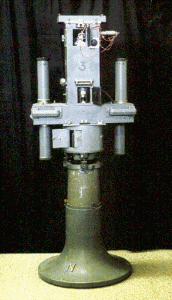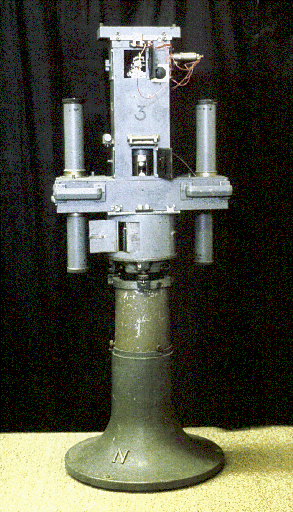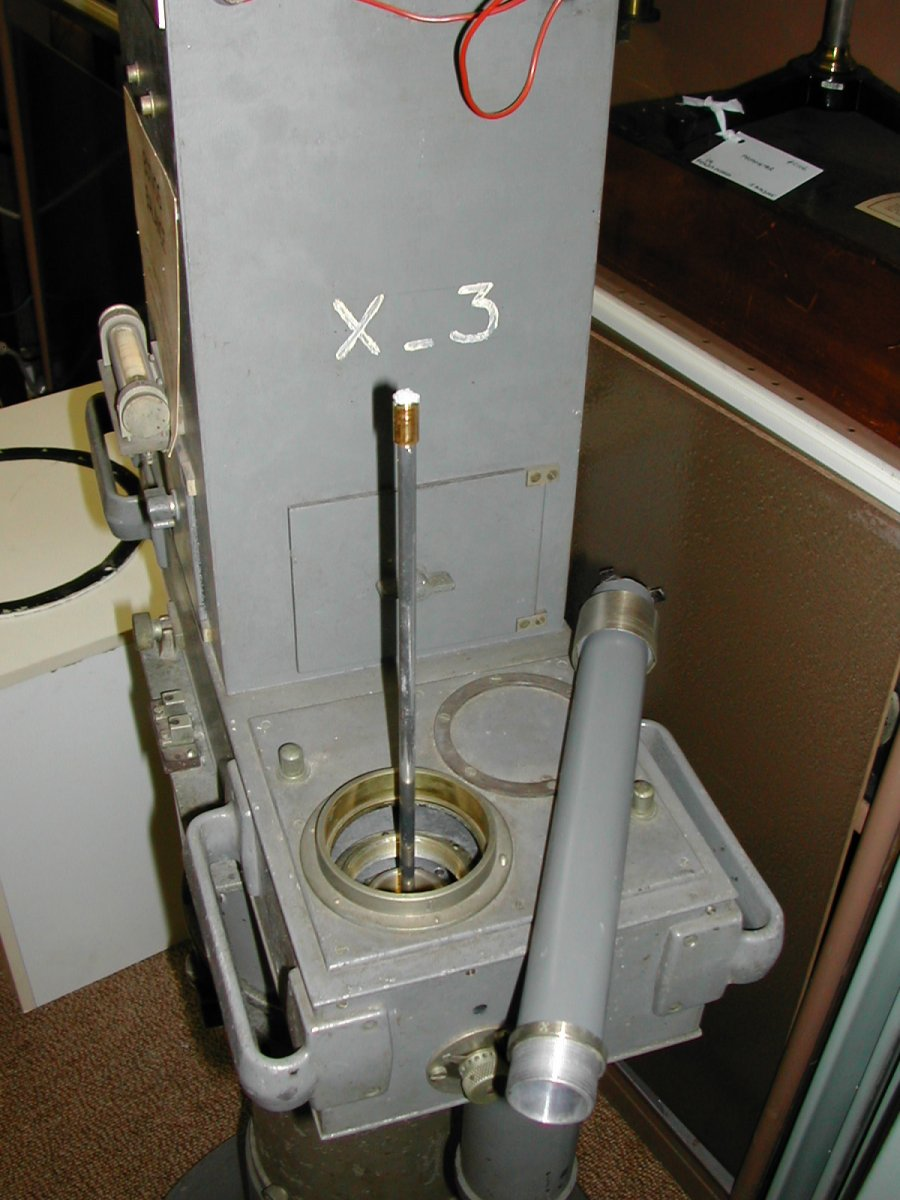ID:
31
Maker's Name:
Askaniawerke AG, Berlin
Where made:
Germany
circa 1920
Dimensions:
80 × 80 × 150 cmConsists of a torsion balance with two platinum masses suspended at different heights by a fixed platinum-iridium wire. Protected by a triple walled torsion chest which may be turned around the axis of the entire instrument and adjusted in any azimuth on a horizontally divided circle. Mechanism for measuring is dominantly clockwork, also enclosed within torsion chest. Developed in 1888 by Professor Lorand Eötvös, the Eötvös Balance was used extensively until the improvement in the accuracy of gravity meters in 1936. The deviation of the Earth's surface from a sphere produces components of the gravity force in the horizontal plane. This turns a suspended beam in the direction of the smallest principle curvature of the Earth's surface. The wire will twist and the moment of rotation depends on the magnitude and direction of the horizontal directing force of gravity. The wire is then turned back by an elastic force produced by the torsion of the wire. The two moments of rotation equilibrate the beam and thus (using the angle of torsion and moment of torsion of the wire) the moment of rotation of gravity can be determined. As the lines of force of gravitation curve downwards in the direction of the gradient the forces of gravitation are not parallel at different heights. Thus the forces affecting the weights suspended from the beam incline towards the axis of rotation at different angles. The components perpendicular to the axis adjust the beam parallel and proportional to the gradient of gravity. Thus the magnitude and direction of the gravity field can be measured from the inclination and declination of the beam suspending the two platinum masses. In use the whole device is rotated by clockwork and measurements are recorded on a photographic plate. Several hours are needed to measure the gradient at one point.

B.S.

••